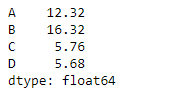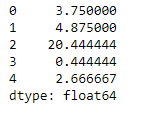Skip to content
Related Articles
Python | Pandas dataframe.mad()
• Last Updated : 19 Nov, 2018

Python is a great language for doing data analysis, primarily because of the fantastic ecosystem of data-centric python packages. Pandas is one of those packages and makes importing and analyzing data much easier.

Pandas` dataframe.mad()` function return the mean absolute deviation of the values for the requested axis. The mean absolute deviation of a dataset is the average distance between each data point and the mean. It gives us an idea about the variability in a dataset.

Syntax: DataFrame.mad(axis=None, skipna=None, level=None)

Parameters :
axis : {index (0), columns (1)}
skipna : Exclude NA/null values when computing the result
level : If the axis is a MultiIndex (hierarchical), count along a particular level, collapsing into a Series
numeric_only : Include only float, int, boolean columns. If None, will attempt to use everything, then use only numeric data. Not implemented for Series.

Returns : mad : Series or DataFrame (if level specified)

Example #1: Use `mad()` function to find the mean absolute deviation of the values over the index axis.

 `# importing pandas as pd``import` `pandas as pd`` ` `# Creating the dataframe ``df ``=` `pd.DataFrame({``"A"``:[``12``, ``4``, ``5``, ``44``, ``1``],``                   ``"B"``:[``5``, ``2``, ``54``, ``3``, ``2``], ``                   ``"C"``:[``20``, ``16``, ``7``, ``3``, ``8``],``                   ``"D"``:[``14``, ``3``, ``17``, ``2``, ``6``]})`` ` `# Print the dataframe``df`Let’s use the `dataframe.mad()` function to find the mean absolute deviation.

 `# find the mean absolute deviation ``# over the index axis``df.mad(axis ``=` `0``)`

Output :Example #2: Use `mad()` function to find the mean absolute deviation of values over the column axis which is having some `Na` values in it.

 `# importing pandas as pd``import` `pandas as pd`` ` `# Creating the dataframe ``df ``=` `pd.DataFrame({``"A"``:[``12``, ``4``, ``5``, ``None``, ``1``],``                   ``"B"``:[``7``, ``2``, ``54``, ``3``, ``None``],``                   ``"C"``:[``20``, ``16``, ``11``, ``3``, ``8``], ``                   ``"D"``:[``14``, ``3``, ``None``, ``2``, ``6``]})`` ` `# To find the mean absolute deviation``# skip the Na values when finding the mad value``df.mad(axis ``=` `1``, skipna ``=` `True``)`

Output :Attention geek! Strengthen your foundations with the Python Programming Foundation Course and learn the basics.

To begin with, your interview preparations Enhance your Data Structures concepts with the Python DS Course. And to begin with your Machine Learning Journey, join the Machine Learning – Basic Level Course

My Personal Notes arrow_drop_up